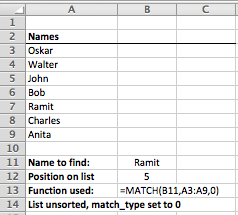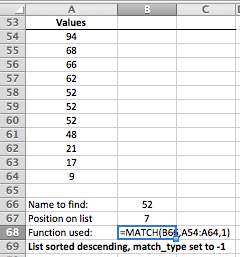# Use the MATCH function in Excel to find the position of a value in a list

The MATCH() function allows you to find the relative position of a value in a list in Excel. For example, in a list of weekdays starting with Monday first, MATCH() would return a value of 3 for Wednesday. This lesson explains how to use the MATCH() function in Microsoft Excel, explains where you might use it, and provides a real world example of the MATCH() function in action.

## MATCH() Function Syntax

The MATCH() function has the following syntax:

=MATCH(lookup_value,lookup_array,match_type)

• lookup_value is the value you want to find in the list. It is required for the function to work.
• lookup_array is the range of cells that contain the list. It is required for the function to work.
• match_type is an optional value that defines the type of match you are looking for. It can have three possible values, -1, 0 and 1. If you leave it out Excel assumes a value of 1.

The MATCH function finds the position of your lookup_value in the range of cells you're looking in (the lookup_array). It's important to note that if your list doesn't include the lookup_value, the MATCH function will return a #NA error.

## Crucial - understanding the match_type parameter

Understanding the match_type parameter is the key to using the MATCH function effectively.

Although match_type is optional, I recommend you always set it to the correct value when using the MATCH function. Otherwise your MATCH functions won't always return the results you expect.

• A match_type value of 1 should be used when your list is sorted in ascending order (smallest to largest).
• If your list contains your lookup_value more than once, MATCH will return the position of the last instance of that value.
• If your list is not sorted you will sometimes get the right answer, but sometimes you'll get a #N/A error.
• If your list doesn't contain your lookup_value, MATCH will return the position of the next largest value.
• If you don't supply a match_type, Excel will automatically use 1 as the match_type.
• A match_type value of 0 means your list doesn't need to be sorted.
• MATCH will look for an exact match for your lookup_value in the list
• MATCH will return the position of the first occurrence of the lookup_value it finds, even if there are duplicates in the list
• Important - MATCH will return a #N/A error if the lookup_value is not in the list.
• A match_type value of -1 should be used when your list is sorted in descending order (largest to smallest).
• If your list is not sorted you will sometimes get the right answer, but sometimes you'll get a #N/A error.
• If your list contains your lookup_value more than once, MATCH will return the position of the last instance in the list of that value.
• If your list doesn't contain your lookup_value, MATCH will return the position of the next smallest value.

## Using the MATCH function in Excel - find a match in a list without duplicates

This example looks at how to use the MATCH function if your list doesn't contain any duplicates.

• The data for this example is a list of people's names. The list is not sorted alphabetically.
• We want to find where a particular person appears in the list. In this case, we know each person only appears once.
• In this situation, you should set match_type to 0. If you don't, Excel will set match_type to 1, and your formula is likely to return the wrong result.• In the example above, B12 contains the MATCH function, which returns a value of 5 since Ramit is 4th in the list.
• Note that you will get a #N/A result if you enter a name into B11 that doesn't exist in the list.
• B13 shows us the MATCH function as it was entered:
• The value to find a match for is in B11. We could also have entered Ramit into the MATCH function direcly as the lookup value rather than referencing B11.
• The range of cells to look up is A3:A9, i.e. the cells containing our list of names.
• We have set the match_type parameter to 0 because each name appears only once.

## The MATCH function in action - finding a match in a list with duplicates

MATCH gets more complicated when you have lists that contain duplicate values since MATCH can only return one value. In other words, even if you have the value you are looking for appears 3 times, MATCH can only give you the position of one of those values.

### Using MATCH in an unsorted list that contains duplicate values

• If your list contains duplicates but is not sorted, the most reliable way to use MATCH is to set match_type to 0.
• This will return the position of the first instance of match_type on your list.
• Also, it will only return a result if there is an exact match. Setting match_type to 1 or -1 when your list is not sorted will return unpredictable and sometimes incorrect results.
• In this example, we have list of numbers that is not sorted and contains duplicate values. We want to find the position of a certain number on that list• In the example above, we are looking for the position of 52 in the list. As you can see, 52 is repeated three times in the list.
• The position returned by the MATCH function in this example is 6 because this is the first instance of 52 that MATCH encountered (when starting from the list and working down). The other two values are ignored by the MATCH function.
• This is a very important fact to remember. MATCH can only find one instance of a value in a list. It will not let you look specifically for the second or third instances of a value in a list.

### Using MATCH in an list sorted in ascending order with duplicate values

• If your list is sorted in ascending order, you should set match_type to 1.
• You can also leave the match_type value out altogether, since Excel will assume a value for 1 if match_type is not present.
• The following example uses the same set of numbers as the previous example:• In this example, note that the list is sorted and that 52 occupies positions 5, 6 and 7 in the list.
• The MATCH function, with match_type set to 1, has returned 7. This is because the last instance of 52 on the list is in position 7.
• Once again, remember that MATCH can only return one value from the list.
• If you want MATCH to return the position of the first instance of 52, you could set match_type to 0.
• There is no reliable way to get MATCH to return the position of the second instance of 52 in the list.

### Using MATCH in an list sorted in descending order with duplicate values

• If your list is sorted in descending order, you should set match_type to -1.
• The following example uses the same set of numbers as the previous two examples:• In this example, note that the list is sorted and that 52 occupies positions 5, 6 and 7 in the list.
• The MATCH function, with match_type set to -1, has returned 7. As with the previous example, this is because the last instance of 52 on the list is in position 7.
• Once again, remember that MATCH can only return one value from the list.
• If you want MATCH to return the position of the first instance of 52, you could set match_type to 0.
• There is no reliable way to get MATCH to return the position of the second instance of 52 in the list.

## Summary of the MATCH function

MATCH performs a very specific and somewhat limited function in Excel. If you are planning to use MATCH in your spreadsheets, make sure you understand the job that match_type does in determining how the MATCH function will decide what result to return. It is also a good idea to include match_type when you use the MATCH function even if you don't need to. That avoids mistakes, and makes troubleshooting easier.

## Our Comment Policy.

Add a comment to this lesson

### How to find second, third, etc

You wrote:
"There is no reliable way to get MATCH to return the position of the second instance of 52 in the list."

[I am discussing only the situation with match_type = 1. That is, the list is assumed to be sorted in ascending order.]

This may be true only if you are limiting yourself to worksheet functions. If you drop into Visual Basic, it is a snap.

You find a match, not necessarily the first one, but any one.
Then you run up the values until you find the first non-match. Now move down one at a time and count matches until you get to the particular match you want, or run out of them.

### Need to get value in a raw, when entering another value

Hi,
I have my Employee details in sheet2, i.e. name, E-code, designation.

When I enter one name in sheet1, I need the particular Employee's E-code to be displayed in the next cell of that particular raw.

Thanks to someone who would help in this.

Amar Lopez

### above question

You enter the name at Sheet1, the name should be same and unique of the Sheet2 emp information.
Go to next cell of name at Sheet 2 then enter the following formula
B2=VLOOKUP(A2,Sheet2!A2:B7,2,0)
Here A2 = given Name
B2= system will give emp code
A2:B7 at Sheet 2 is the list of emp information.

### Error in explanation of lookup_value=1

If lookup_value = 1 in a list sorted in ascending order, then MATCH returns the position of the value that is smaller than or equal to the lookup_value.
So, your text is wrong, as it states that is will return the next larger value in such case.

sdsf